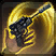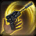# Hot StreakConditions

## Used by

Used by
• apc.smuggler.scoundrel.base

## Related effects, buffs and debuffs

• [15s]Hot Streak
Mastery increased.

 Slot: Buff Duration: 15s Tick rate: does not tick # occurrences: 1
• When effect is applied
• When effect is refreshed

Perform the following actions:

• Play appearance epp . agent . hot_streak . cast_instant, dependent on calling effect
• On Apply

Perform the following actions:

• On Apply

Perform the following actions:

• Modify Cooldown
- Is Percentage = (bool) true
- Tag = (string)
- Tags = (string)
- Ability Spec = (int) 16140979154918576240
- Amount Min = (float) 0
- Amount = (float) -1
• Modify Cooldown
- Is Percentage = (bool) true
- Tag = (string)
- Tags = (string)
- Ability Spec = (int) 16141040506483181958
- Amount Min = (float) 0
- Amount = (float) -1
• Modify Cooldown
- Is Percentage = (bool) true
- Tag = (string)
- Tags = (string)
- Ability Spec = (int) 16140954132768784345
- Amount Min = (float) 0
- Amount = (float) -1
• Modify Cooldown
- Is Percentage = (bool) true
- Tag = (string)
- Tags = (string)
- Ability Spec = (int) 16141127024227488390
- Amount Min = (float) 0
- Amount = (float) -1
• Modify Cooldown
- Is Percentage = (bool) true
- Tag = (string)
- Tags = (string)
- Ability Spec = (int) 16141144605439818307
- Amount Min = (float) 0
- Amount = (float) -1